xMonoidEncyclopedia
In abstract algebra
Abstract algebra
Abstract algebra is the subject area of mathematics that studies algebraic structures, such as groups, rings, fields, modules, vector spaces, and algebras...

, a branch of mathematics
Mathematics
Mathematics is the study of quantity, space, structure, and change. Mathematicians seek out patterns and formulate new conjectures. Mathematicians resolve the truth or falsity of conjectures by mathematical proofs, which are arguments sufficient to convince other mathematicians of their validity...

, a monoid is an algebraic structure
Algebraic structure
In abstract algebra, an algebraic structure consists of one or more sets, called underlying sets or carriers or sorts, closed under one or more operations, satisfying some axioms. Abstract algebra is primarily the study of algebraic structures and their properties...

with a single associative binary operation
Binary operation
In mathematics, a binary operation is a calculation involving two operands, in other words, an operation whose arity is two. Examples include the familiar arithmetic operations of addition, subtraction, multiplication and division....

and an identity element
Identity element
In mathematics, an identity element is a special type of element of a set with respect to a binary operation on that set. It leaves other elements unchanged when combined with them...

. Monoids are studied in semigroup
Semigroup
In mathematics, a semigroup is an algebraic structure consisting of a set together with an associative binary operation. A semigroup generalizes a monoid in that there might not exist an identity element...

theory as they are naturally semigroups with identity. Monoids occur in several branches of mathematics; for instance, they can be regarded as categories
Category (mathematics)
In mathematics, a category is an algebraic structure that comprises "objects" that are linked by "arrows". A category has two basic properties: the ability to compose the arrows associatively and the existence of an identity arrow for each object. A simple example is the category of sets, whose...

with a single object. Thus, they capture the idea of function composition
Function composition
In mathematics, function composition is the application of one function to the results of another. For instance, the functions and can be composed by computing the output of g when it has an argument of f instead of x...

within a set. Monoids are also commonly used in computer science
Computer science
Computer science or computing science is the study of the theoretical foundations of information and computation and of practical techniques for their implementation and application in computer systems...

, both in its foundational aspects and in practical programming. The transition monoid and syntactic monoid
Syntactic monoid
In mathematics and computer science, the syntactic monoid M of a formal language L is the smallest monoid that recognizes the language L.-Syntactic quotient:...

are used in describing finite state machines, whereas trace monoid
Trace monoid
In mathematics and computer science, a trace is a set of strings, wherein certain letters in the string are allowed to commute, but others are not. It generalizes the concept of a string, by not forcing the letters to always be in a fixed order, but allowing certain reshufflings to take place...

s and history monoid
History monoid
In mathematics and computer science, a history monoid is a way of representing the histories of concurrently running computer processes as a collection of strings, each string representing the individual history of a process...

s provide a foundation for process calculi and concurrent computing
Concurrent computing
Concurrent computing is a form of computing in which programs are designed as collections of interacting computational processes that may be executed in parallel...

. Some of the more important results in the study of monoids are the Krohn-Rhodes theorem and the star height problem
Star height problem
The star height problem in formal language theory is the question whether all regular languages can be expressed using regular expressions of limited star height, i.e. with a limited nesting depth of Kleene stars...

. The history of monoids, as well as a discussion of additional general properties, are found in the article on semigroups.

## Definition

A monoid is a set, S, together with a binary operation
Binary operation
In mathematics, a binary operation is a calculation involving two operands, in other words, an operation whose arity is two. Examples include the familiar arithmetic operations of addition, subtraction, multiplication and division....

“•” that satisfies the following three axioms:

Closure: For all a, b in S, the result of the operation a • b is also in S.
Associativity: For all a, b and c in S, the equation (a • b) • c = a • (b • c) holds.
Identity element: There exists an element e in S, such that for all elements a in S, the equation e • a = a • e = a holds.
And in mathematical notation we can write these as
• Closure: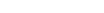,
• Associativity: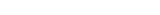and
• Identity element: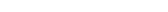.

More compactly, a monoid is a semigroup
Semigroup
In mathematics, a semigroup is an algebraic structure consisting of a set together with an associative binary operation. A semigroup generalizes a monoid in that there might not exist an identity element...

with an identity element
Identity element
In mathematics, an identity element is a special type of element of a set with respect to a binary operation on that set. It leaves other elements unchanged when combined with them...

. It can also be thought of as a magma
Magma (algebra)
In abstract algebra, a magma is a basic kind of algebraic structure. Specifically, a magma consists of a set M equipped with a single binary operation M \times M \rightarrow M....

with associativity and identity. A monoid with invertibility
Inverse element
In abstract algebra, the idea of an inverse element generalises the concept of a negation, in relation to addition, and a reciprocal, in relation to multiplication. The intuition is of an element that can 'undo' the effect of combination with another given element...

property is a group
Group (mathematics)
In mathematics, a group is an algebraic structure consisting of a set together with an operation that combines any two of its elements to form a third element. To qualify as a group, the set and the operation must satisfy a few conditions called group axioms, namely closure, associativity, identity...

.

The symbol for the binary operation is commonly omitted; for example the monoid axioms require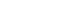and. This does not necessarily mean the variables are numbers being multiplied, any operation or elements may be used if they are well defined.

### Generators and submonoids

A submonoid of a monoid M is a subset N of M containing the unit element, and such that, if x,y ∈ N then x · y ∈ N. It is then clear that N is itself a monoid, under the binary operation induced by that of M. Equivalently, a submonoid is a subset N such that N=N*, where the superscript * is the Kleene star
Kleene star
In mathematical logic and computer science, the Kleene star is a unary operation, either on sets of strings or on sets of symbols or characters. The application of the Kleene star to a set V is written as V*...

: the set is closed under composition or concatenation of its elements. For any subset N of M, the monoid N* is the smallest monoid that contains N.

A subset N is said to be a generator of M if and only if M=N*. If there is a finite generator of M, then M is said to be finitely generated.

### Commutative monoid

A monoid whose operation is commutative is called a commutative monoid (or, less commonly, an abelian monoid). Commutative monoids are often written additively. Any commutative monoid is endowed with its algebraic preorder
Preorder
In mathematics, especially in order theory, preorders are binary relations that are reflexive and transitive.For example, all partial orders and equivalence relations are preorders...

ing ≤, defined by x ≤ y if and only if there exists z such that x + z = y. An order-unit of a commutative monoid M is an element u of M such that for any element x of M, there exists a positive integer n such that x ≤ nu. This is often used in case M is the positive cone
Ordered group
In abstract algebra, a partially-ordered group is a group equipped with a partial order "≤" that is translation-invariant; in other words, "≤" has the property that, for all a, b, and g in G, if a ≤ b then a+g ≤ b+g and g+a ≤ g+b.An element x of G is called positive element if 0 ≤ x...

of a partially ordered
Partially ordered set
In mathematics, especially order theory, a partially ordered set formalizes and generalizes the intuitive concept of an ordering, sequencing, or arrangement of the elements of a set. A poset consists of a set together with a binary relation that indicates that, for certain pairs of elements in the...

abelian group
Abelian group
In abstract algebra, an abelian group, also called a commutative group, is a group in which the result of applying the group operation to two group elements does not depend on their order . Abelian groups generalize the arithmetic of addition of integers...

G, in which case we say that u is an order-unit of G.

### Partially commutative monoid

A monoid for which the operation is commutative for some, but not all elements is a trace monoid
Trace monoid
In mathematics and computer science, a trace is a set of strings, wherein certain letters in the string are allowed to commute, but others are not. It generalizes the concept of a string, by not forcing the letters to always be in a fixed order, but allowing certain reshufflings to take place...

; trace monoids commonly occur in the theory of concurrent computation.

## Examples

• Every singleton set {x} gives rise to a particular one-element (trivial) monoid. The monoid axioms require that x*x = x in this case.
• Every group
Group (mathematics)
In mathematics, a group is an algebraic structure consisting of a set together with an operation that combines any two of its elements to form a third element. To qualify as a group, the set and the operation must satisfy a few conditions called group axioms, namely closure, associativity, identity...

is a monoid and every abelian group
Abelian group
In abstract algebra, an abelian group, also called a commutative group, is a group in which the result of applying the group operation to two group elements does not depend on their order . Abelian groups generalize the arithmetic of addition of integers...

a commutative monoid.
• Every bounded semilattice
Semilattice
In mathematics, a join-semilattice is a partially ordered set which has a join for any nonempty finite subset. Dually, a meet-semilattice is a partially ordered set which has a meet for any nonempty finite subset...

is an idempotent commutative monoid.
• In particular, any bounded lattice
Lattice (order)
In mathematics, a lattice is a partially ordered set in which any two elements have a unique supremum and an infimum . Lattices can also be characterized as algebraic structures satisfying certain axiomatic identities...

can be endowed with both a meet- and a join- monoid structure. The identity elements are the lattice's top and its bottom, respectively. Being lattices, Heyting
Heyting algebra
In mathematics, a Heyting algebra, named after Arend Heyting, is a bounded lattice equipped with a binary operation a→b of implication such that ∧a ≤ b, and moreover a→b is the greatest such in the sense that if c∧a ≤ b then c ≤ a→b...

and Boolean algebra are endowed with these monoid structures.
• Any semigroup
Semigroup
In mathematics, a semigroup is an algebraic structure consisting of a set together with an associative binary operation. A semigroup generalizes a monoid in that there might not exist an identity element...

S may be turned into a monoid simply by adjoining an element e not in S and defining e*s = s = s*e for all s ∈ S. This conversion of any semigroup to the monoid is done by the free functor between the category of semigroups and the category of monoids .
• Thus, an idempotent monoid (sometimes known as find-first) may be formed by adjoining an identity element e to the left zero semigroup over a set S. The opposite monoid (sometimes called find-last) is formed from the right zero semigroup over S.
• Adjoin an identity e to the left-zero semigroup with two elements {lt; gt}. Then the resulting idempotent monoid {lt; e; gt} models the lexicographical order
Lexicographical order
In mathematics, the lexicographic or lexicographical order, , is a generalization of the way the alphabetical order of words is based on the alphabetical order of letters.-Definition:Given two partially ordered sets A and B, the lexicographical order on...

of a sequence given the orders of its elements, with e representing equality.
• The natural number
Natural number
In mathematics, the natural numbers are the ordinary whole numbers used for counting and ordering . These purposes are related to the linguistic notions of cardinal and ordinal numbers, respectively...

s, N, form a commutative monoid under addition (identity element zero
0 (number)
0 is both a numberand the numerical digit used to represent that number in numerals.It fulfills a central role in mathematics as the additive identity of the integers, real numbers, and many other algebraic structures. As a digit, 0 is used as a placeholder in place value systems...

), or multiplication (identity element one). A submonoid of N under addition is called a numerical monoid.
• The positive integers, N-{0}, form a commutative monoid under multiplication (identity element one).
• The elements of any unital ring, with addition or multiplication as the operation.
• The integer
Integer
The integers are formed by the natural numbers together with the negatives of the non-zero natural numbers .They are known as Positive and Negative Integers respectively...

s, rational number
Rational number
In mathematics, a rational number is any number that can be expressed as the quotient or fraction a/b of two integers, with the denominator b not equal to zero. Since b may be equal to 1, every integer is a rational number...

s, real number
Real number
In mathematics, a real number is a value that represents a quantity along a continuum, such as -5 , 4/3 , 8.6 , √2 and π...

s or complex number
Complex number
A complex number is a number consisting of a real part and an imaginary part. Complex numbers extend the idea of the one-dimensional number line to the two-dimensional complex plane by using the number line for the real part and adding a vertical axis to plot the imaginary part...

s, with addition or multiplication as operation.
• The set of all n by n matrices
Matrix (mathematics)
In mathematics, a matrix is a rectangular array of numbers, symbols, or expressions. The individual items in a matrix are called its elements or entries. An example of a matrix with six elements isMatrices of the same size can be added or subtracted element by element...

over a given ring, with matrix addition
In mathematics, matrix addition is the operation of adding two matrices by adding the corresponding entries together. However, there are other operations which could also be considered as a kind of addition for matrices, the direct sum and the Kronecker sum....

or matrix multiplication
Matrix multiplication
In mathematics, matrix multiplication is a binary operation that takes a pair of matrices, and produces another matrix. If A is an n-by-m matrix and B is an m-by-p matrix, the result AB of their multiplication is an n-by-p matrix defined only if the number of columns m of the left matrix A is the...

as the operation.
• The set of all finite strings
String (computer science)
In formal languages, which are used in mathematical logic and theoretical computer science, a string is a finite sequence of symbols that are chosen from a set or alphabet....

over some fixed alphabet Σ forms a monoid with string concatenation as the operation. The empty string
Empty string
In computer science and formal language theory, the empty string is the unique string of length zero. It is denoted with λ or sometimes Λ or ε....

serves as the identity element. This monoid is denoted Σ and is called the free monoid over Σ.
• Given any monoid M, the opposite monoid Mop has the same carrier set and identity element as M, and its operation is defined by x *op y = y * x. Any commutative monoid is the opposite monoid of itself.
• Given two sets M and N endowed with monoid structure (or, in general, any finite number of monoids, M1, ..., Mk), their cartesian product
Cartesian product
In mathematics, a Cartesian product is a construction to build a new set out of a number of given sets. Each member of the Cartesian product corresponds to the selection of one element each in every one of those sets...

M × N is also a monoid (respectivelly, M1 × ... × Mk). The associative operation and the identity element are defined pairwise.
• Fix a monoid M. The set of all functions from a given set to M is also a monoid. The identity element is a constant function
Constant function
In mathematics, a constant function is a function whose values do not vary and thus are constant. For example the function f = 4 is constant since f maps any value to 4...

mapping any value to the identity of M; the associative operation is defined pointwise
Pointwise
In mathematics, the qualifier pointwise is used to indicate that a certain property is defined by considering each value f of some function f. An important class of pointwise concepts are the pointwise operations — operations defined on functions by applying the operations to function values...

.
• Fix a monoid M with the operation * and identity element e, and consider its power set P(M) consisting of all subset
Subset
In mathematics, especially in set theory, a set A is a subset of a set B if A is "contained" inside B. A and B may coincide. The relationship of one set being a subset of another is called inclusion or sometimes containment...

s of M. A binary operation for such subsets can be defined by S * T = {s * t : s in S and t in T}. This turns P(M) into a monoid with identity element {e}. In the same way the power set of a group G is a monoid under the product of group subsets.
• Let S be a set. The set of all functions S → S forms a monoid under function composition
Function composition
In mathematics, function composition is the application of one function to the results of another. For instance, the functions and can be composed by computing the output of g when it has an argument of f instead of x...

. The identity is just the identity function
Identity function
In mathematics, an identity function, also called identity map or identity transformation, is a function that always returns the same value that was used as its argument...

. It is also called the full transformation monoid of S. If S is finite with n elements, the monoid of functions on S is finite with nn elements.
• Generalizing the previous example, let C be a category
Category (mathematics)
In mathematics, a category is an algebraic structure that comprises "objects" that are linked by "arrows". A category has two basic properties: the ability to compose the arrows associatively and the existence of an identity arrow for each object. A simple example is the category of sets, whose...

and X an object in C. The set of all endomorphism
Endomorphism
In mathematics, an endomorphism is a morphism from a mathematical object to itself. For example, an endomorphism of a vector space V is a linear map ƒ: V → V, and an endomorphism of a group G is a group homomorphism ƒ: G → G. In general, we can talk about...

s of X, denoted EndC(X), forms a monoid under composition of morphism
Morphism
In mathematics, a morphism is an abstraction derived from structure-preserving mappings between two mathematical structures. The notion of morphism recurs in much of contemporary mathematics...

s. For more on the relationship between category theory and monoids see below.
• The set of homeomorphism
Homeomorphism
In the mathematical field of topology, a homeomorphism or topological isomorphism or bicontinuous function is a continuous function between topological spaces that has a continuous inverse function. Homeomorphisms are the isomorphisms in the category of topological spaces—that is, they are...

classes
Class (set theory)
In set theory and its applications throughout mathematics, a class is a collection of sets which can be unambiguously defined by a property that all its members share. The precise definition of "class" depends on foundational context...

of compact surfaces with the connected sum
Connected sum
In mathematics, specifically in topology, the operation of connected sum is a geometric modification on manifolds. Its effect is to join two given manifolds together near a chosen point on each...

. Its unit element is the class of the ordinary 2-sphere. Furthermore, if a denotes the class of the torus, and b denotes the class of the projective plane, then every element c of the monoid has a unique expression the form c=na+mb where n is the integer ≥ 0 and m=0,1, or 2. We have 3b=a+b.
• Let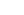be a cyclic monoid of order n, that is,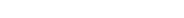. Then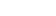for some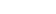. In fact, each such k gives a distinct monoid of order n, and every cyclic monoid is isomorphic to one of these.

Moreover, f can be considered as a function on the points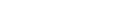given by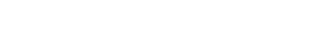or, equivalentlyMultiplication of elements inis then given by function composition.

Note also that when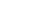then the function f is a permutation ofand gives the unique cyclic group
Cyclic group
In group theory, a cyclic group is a group that can be generated by a single element, in the sense that the group has an element g such that, when written multiplicatively, every element of the group is a power of g .-Definition:A group G is called cyclic if there exists an element g...

of order n.

## Properties

In a monoid, one can define positive integer powers of an element x : x1=x, and xn=x*...*x (n times) for n>1 . The rule of powers xn+p=xn * xp is obvious.

Directly from the definition, one can show that the identity element e is unique. Then, for any x , one can set x0=e and the rule of powers is still true with nonnegative exponents.

It is possible to define invertible elements
Inverse element
In abstract algebra, the idea of an inverse element generalises the concept of a negation, in relation to addition, and a reciprocal, in relation to multiplication. The intuition is of an element that can 'undo' the effect of combination with another given element...

: an element x is called invertible if there exists an element y such that x*y = e and y*x = e. The element y is called the inverse of x . If y and z are inverses of x, then by associativity y = (zx)y = z(xy) = z. Thus inverses, if they exist, are unique.

If y is the inverse of x , one can define negative powers of x by setting x−1=y and x−n=y*...*y (n times) for n>1 . And the rule of exponents is still verified for all n,p rational integers. This is why the inverse of x is usually written x−1. The set of all invertible elements in a monoid M, together with the operation *, forms a group
Group (mathematics)
In mathematics, a group is an algebraic structure consisting of a set together with an operation that combines any two of its elements to form a third element. To qualify as a group, the set and the operation must satisfy a few conditions called group axioms, namely closure, associativity, identity...

. In that sense, every monoid contains a group (if only the trivial one consisting of the identity alone).

However, not every monoid sits inside a group. For instance, it is perfectly possible to have a monoid in which two elements a and b exist such that a*b = a holds even though b is not the identity element. Such a monoid cannot be embedded in a group, because in the group we could multiply both sides with the inverse of a and would get that b = e, which isn't true. A monoid (M,*) has the cancellation property
Cancellation property
In mathematics, the notion of cancellative is a generalization of the notion of invertible.An element a in a magma has the left cancellation property if for all b and c in M, a * b = a * c always implies b = c.An element a in a magma has the right cancellation...

(or is cancellative) if for all a, b and c in M, a*b = a*c always implies b = c and b*a = c*a always implies b = c. A commutative monoid with the cancellation property can always be embedded in a group via the Grothendieck construction
Grothendieck group
In mathematics, the Grothendieck group construction in abstract algebra constructs an abelian group from a commutative monoid in the best possible way...

. That's how the additive group of the integers (a group with operation +) is constructed from the additive monoid of natural numbers (a commutative monoid with operation + and cancellation property). However, a non-commutative cancellative monoid need not be embeddable in a group.

If a monoid has the cancellation property and is finite, then it is in fact a group. Proof: Fix an element x in the monoid. Since the monoid is finite, xn = xm for some m > n > 0. But then, by cancellation we have that xm-n = e where e is the identity. Therefore x * xm-n-1 = e, so x has an inverse.

The right- and left-cancellative elements of a monoid each in turn form a submonoid (i.e. obviously include the identity and not so obviously are closed under the operation). This means that the cancellative elements of any commutative monoid can be extended to a group.

An inverse monoid is a monoid where for every a in M, there exists a unique a-1 in M such that a=a*a-1*a and a-1=a-1*a*a−1. If an inverse monoid is cancellative, then it is a group.

## Acts and operator monoids

Let M be a monoid, with the binary operation denoted by “•” and the identity element denoted by e. Then a (left) M-act (or left act over M) is a set X together with an operation ⋅ : M × X → X which is compatible with the monoid structure as follows:
• for all x in X: e ⋅ x = x;
• for all a, b in M and x in X: a ⋅ (b ⋅ x) = (a • b) ⋅ x.

This is the analogue in monoid theory of a (left) group action
Group action
In algebra and geometry, a group action is a way of describing symmetries of objects using groups. The essential elements of the object are described by a set, and the symmetries of the object are described by the symmetry group of this set, which consists of bijective transformations of the set...

. Right M-acts are defined in a similar way. A monoid with an act is also known as an operator monoid. Important examples include transition systems of semiautomata. A transformation semigroup
Transformation semigroup
In algebra and theoretical computer science, an action or act of a semigroup on a set is a rule which associates to each element of the semigroup a transformation of the set in such a way that the product of two elements of the semigroup is associated with the composite of the two corresponding...

can be made into an operator monoid by adjoining the identity transformation.

## Monoid homomorphisms

A homomorphism
Homomorphism
In abstract algebra, a homomorphism is a structure-preserving map between two algebraic structures . The word homomorphism comes from the Greek language: ὁμός meaning "same" and μορφή meaning "shape".- Definition :The definition of homomorphism depends on the type of algebraic structure under...

between two monoids (M,*) and (M′,•) is a function f : M → M′ such that
• f(x*y) = f(x)•f(y) for all x, y in M
• f(e) = e′

where e and e′ are the identities on M and M′ respectively. Monoid homomorphisms are sometimes simply called monoid morphisms.

Not every semigroup homomorphism is a monoid homomorphism since it may not preserve the identity. Contrast this with the case of group homomorphism
Group homomorphism
In mathematics, given two groups and , a group homomorphism from to is a function h : G → H such that for all u and v in G it holds that h = h \cdot h...

s: the axioms of group theory
Group theory
In mathematics and abstract algebra, group theory studies the algebraic structures known as groups.The concept of a group is central to abstract algebra: other well-known algebraic structures, such as rings, fields, and vector spaces can all be seen as groups endowed with additional operations and...

ensure that every semigroup homomorphism between groups preserves the identity. For monoids this isn't always true and it is necessary to state it as a separate requirement.

A bijective monoid homomorphism is called a monoid isomorphism
Isomorphism
In abstract algebra, an isomorphism is a mapping between objects that shows a relationship between two properties or operations.  If there exists an isomorphism between two structures, the two structures are said to be isomorphic.  In a certain sense, isomorphic structures are...

. Two monoids are said to be isomorphic if there is an isomorphism between them.

## Equational presentation

Monoids may be given a presentation, much in the same way that groups can be specified by means of a group presentation. One does this by specifying a set of generators Σ, and a set of relations on the free monoid Σ. One does this by extending (finite) binary relation
Binary relation
In mathematics, a binary relation on a set A is a collection of ordered pairs of elements of A. In other words, it is a subset of the Cartesian product A2 = . More generally, a binary relation between two sets A and B is a subset of...

s on Σ to monoid congruences, and then constructing the quotient monoid, as above.

Given a binary relation R ⊂ Σ × Σ, one defines its symmetric closure as R ∪ R−1. This can be extended to a symmetric relation E ⊂ Σ × Σ by defining x ~E y if and only if x = sut and y = svt for some strings u, v, s, t ∈ Σ with (u,v) ∈ R ∪ R−1. Finally, one takes the reflexive and transitive closure of E, which is then a monoid congruence.

In the typical situation, the relation R is simply given as a set of equations, so that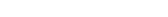. Thus, for example,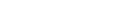is the equational presentation for the bicyclic monoid, and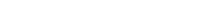is the plactic monoid
Plactic monoid
In mathematics, the plactic monoid is the monoid of all words in the alphabet of positive integers modulo Knuth equivalence. Its elements can be identified with semistandard Young tableaux...

of degree 2 (it has infinite order). Elements of this plactic monoid may be written as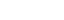for integers i, j, k, as the relations show that ba commutes with both a and b.

## Relation to category theory

Monoids can be viewed as a special class of categories
Category theory
Category theory is an area of study in mathematics that examines in an abstract way the properties of particular mathematical concepts, by formalising them as collections of objects and arrows , where these collections satisfy certain basic conditions...

. Indeed, the axioms required of a monoid operation are exactly those required of morphism
Morphism
In mathematics, a morphism is an abstraction derived from structure-preserving mappings between two mathematical structures. The notion of morphism recurs in much of contemporary mathematics...

composition when restricted to the set of all morphisms whose source and target is a given object. That is,
A monoid is, essentially, the same thing as a category with a single object.

More precisely, given a monoid (M,*), one can construct a small category with only one object and whose morphisms are the elements of M. The composition of morphisms is given by the monoid operation *.

Likewise, monoid homomorphisms are just functor
Functor
In category theory, a branch of mathematics, a functor is a special type of mapping between categories. Functors can be thought of as homomorphisms between categories, or morphisms when in the category of small categories....

s between single object categories. So this construction gives an equivalence
Equivalence of categories
In category theory, an abstract branch of mathematics, an equivalence of categories is a relation between two categories that establishes that these categories are "essentially the same". There are numerous examples of categorical equivalences from many areas of mathematics...

between the category of (small) monoids Mon and a full subcategory of the category of (small) categories Cat. Similarly, the category of groups
Category of groups
In mathematics, the category Grp has the class of all groups for objects and group homomorphisms for morphisms. As such, it is a concrete category...

is equivalent to another full subcategory of Cat.

In this sense, category theory can be thought of as an extension of the concept of a monoid. Many definitions and theorems about monoids can be generalised to small categories with more than one object. For example, a quotient of a category with one object is just a quotient monoid.

Monoids, just like other algebraic structures, also form their own category, Mon, whose objects are monoids and whose morphisms are monoid homomorphisms.

There is also a notion of monoid object
Monoid (category theory)
In category theory, a monoid in a monoidal category is an object M together with two morphisms* \mu : M\otimes M\to M called multiplication,* and \eta : I\to M called unit,...

which is an abstract definition of what is a monoid in a category. A monoid object in Set
Category of sets
In the mathematical field of category theory, the category of sets, denoted as Set, is the category whose objects are sets. The arrows or morphisms between sets A and B are all functions from A to B...

is just a monoid.

## Monoids in computer science

In computer science, many abstract data types can be endowed with a monoid structure. In a common pattern, a sequence
Sequence
In mathematics, a sequence is an ordered list of objects . Like a set, it contains members , and the number of terms is called the length of the sequence. Unlike a set, order matters, and exactly the same elements can appear multiple times at different positions in the sequence...

of elements of a monoid is "folded
Fold (higher-order function)
In functional programming, fold – also known variously as reduce, accumulate, compress, or inject – are a family of higher-order functions that analyze a recursive data structure and recombine through use of a given combining operation the results of recursively processing its...

" or "accumulated" to produce a final value. For instance, many iterative algorithms need to update some kind of "running total" at each iteration; this pattern may be elegantly expressed by a monoid operation. Alternatively, the associativity of monoid operations ensures that the operation can be parallelized by employing a prefix sum
Prefix sum
In computer science, the prefix sum, or scan, of a sequence of numbers is a second sequence of numbers , the sums of prefixes of the input sequence:-Parallel algorithm:A prefix sum can be calculated in parallel by the following steps....

or similar algorithm, in order to utilize multiple cores or processors efficiently.

Given a sequence of values of type M with identity elementand associative operation, the fold operation is defined as follows: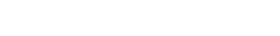Data structure
In computer science, a data structure is a particular way of storing and organizing data in a computer so that it can be used efficiently.Different kinds of data structures are suited to different kinds of applications, and some are highly specialized to specific tasks...

can be 'folded' in a similar way, given a serialization of its elements. For instance, the result of "folding" a binary tree
Binary tree
In computer science, a binary tree is a tree data structure in which each node has at most two child nodes, usually distinguished as "left" and "right". Nodes with children are parent nodes, and child nodes may contain references to their parents. Outside the tree, there is often a reference to...

might differ depending on pre-order vs. post-order tree traversal
Tree traversal
In computer science, tree-traversal refers to the process of visiting each node in a tree data structure, exactly once, in a systematic way. Such traversals are classified by the order in which the nodes are visited...

.